Computer Science Canada Programming C, C++, Java, PHP, Ruby, Turing, VB   Username:   Password:WikiBlogSearchTuringChat RoomMembers
Perfect Circle - Circle Collision DetectionAuthor Message
zylumPosted: Tue Jan 16, 2007 7:13 pm   Post subject: Perfect Circle - Circle Collision DetectionPerfect Circle-Circle Collision Detection

Circular collision detection is a fundamental concept in many types of games. Although there are many different
methods one can use to detect a collision between two circles, the method I am about to describe allows for
accurate collision detection even when the circles are moving at high speeds. Additionally, this method will
allow your program to draw every collision that occurs (if you so wish).

The simplest method to detect a collision is to check if the circles are overlapping. This can be done by
directly applying the Pythagorean Theorem. If The distance between the centers of the two circles is less than or
equal to the sum of the radii of the circles, then a collision has occured. The square root in the distance
formula is unnecessary because the inequality will still hold if we square both sides. Removing the square root
can increase your collision detection especially if you are checking for many collisions.

This method can be satisfactory for many applications but in many cases a more accurate method will be needed. For
instance, what if you require your collision detection algorithm to detect the collisions exactly when they
occur, ie. such that no overlapping occurs? Also if the circles are moving fast enough, this method might not
catch the collision at all! For example, if two circles are close together and moving very quickly towards each
other, in the next frame they could already be past each other. No collision is detected because the collision
occurs in between frames. This problem is not uncommon and is one of the challenges one must overcome when
creating a pool game.Figure 1 - Collision Between Frames

In order to overcome this problem, you need to use a little math. First lets describe the position of a pool ball
that is in motion with no friction acting on it (we will add this later). So the ball will have a certain position
(x, y), and a constant velocity (vy, vy). The ball's new position (x', y'), can be described like so: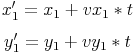Since we will be dealing with more than one ball we use subscripts to describe which ball we are talking about.
The new position is the position of the ball after t time. In our case the unit for t is frames, so if we set
t = 1, then the new position is where the ball will be in the next frame of animation. With these equations, we
can now write a distance equation which, tells us the distance between the centers of the balls. This equation can
be written like so: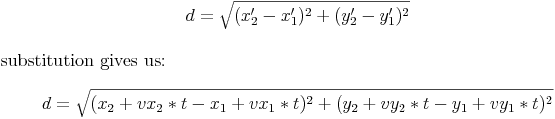Now that we have our distance equation, we can figure out exactly when a collision occurs. All we have to do is
isolate the variable t. This is easier said than done. Using a math program like mathematica or matlab or maple,
you get the equation: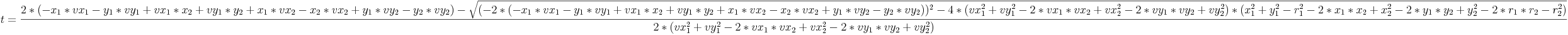Note that since there is a square root in our equation, t may take on two values, one value, or no values at all
and we need to take this into account when we are programming our solution. No solution occurs when there wont be
a collision between the balls, one solution occurs when the balls just skim each other, and two solutions occur
when the balls collide normally. The first solution tells us when the balls initially collide and the second
solution tells us when the balls will touch if they were allowed to pass through each other. Note: A collision in
this case is defined as the balls just touching. If the balls are overlapping, this is not considered a collision.
(this scenerio should never occur anyways)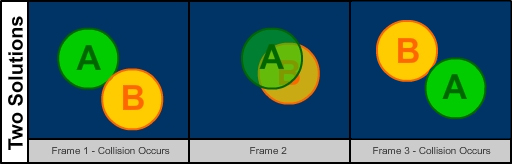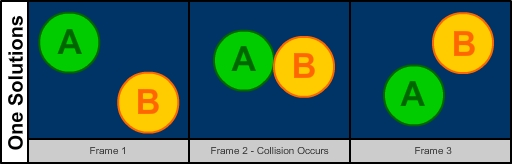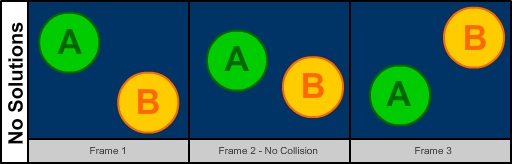Figure 2 - Different Solution Types

Now that we have our formula for time, we can start writing some code. Lets start with writing some code which we
can use to test our collision detection:

 Turing: View.Set ("offscreenonly") type BallData :     record         x, y, vx, vy, r : real     end record /* The amount of delay between frames */ const DELAY := 50 /* We anticipate many balls so we create an array */ var balls : array 1 .. 2 of BallData /* Stores the amount of time untill a collision occurs */ var t : real balls (1).x := 200 balls (1).y := 150 balls (1).vx := 4 balls (1).vy := 4 balls (1).r := 20 balls (2).x := 300 balls (2).y := 250 balls (2).vx := -4 balls (2).vy := -2 balls (2).r := 20 /* Returns the amount of frames untill a collision will occur */ fcn timeToCollision : real     var t : real := maxint     result t end timeToCollision /* Draws all the balls to the screen */ proc drawBalls     for i : 1 .. upper (balls)         Draw.Oval (round (balls (i).x), round (balls (i).y), round (balls (i).r), round (balls (i).r), black)     end for end drawBalls /* Updates all the balls attributes. If a collision Occures in between frames, *  * the balls will be updated to the point of the collision. We return when the *  * collision occurs so that we can adjust the delay in the main loop.          */ fcn updateBalls : real     /* We want to increment by at most one frame */     var t := min (1, timeToCollision)     for i : 1 .. upper (balls)         balls (i).x += balls (i).vx * t         balls (i).y += balls (i).vy * t     end for     result t end updateBalls /* Checks if a collision has occured between any of the balls */ proc checkForCollision     for i : 1 .. upper (balls)         for j : i + 1 .. upper (balls)             if (balls (j).x - balls (i).x) ** 2 + (balls (j).y - balls (i).y) ** 2 <= (balls (i).r + balls (j).r) ** 2 then                 put "Collision has occurd!"                 drawBalls                 View.Update                 delay (500)                 /* Collision reaction code should replace the above four statements. *                  * We will leave collision reaction for another tutorial ;)          */             end if         end for     end for end checkForCollision /* Main animation loop */ loop     drawBalls     checkForCollision     t := updateBalls     View.Update     cls     /* If a collision occurs in between frames, we have to use a shorter delay *      * to keep the animation speed consistent                                  */     Time.DelaySinceLast (round (DELAY * t)) end loop

Listing 1 - Collision Detection Test Program

This program is pretty straight forward. At this point, it is using the simple method for collision detection
mentioned earlier in this tutorial. Notice how a collision is recognized when the balls are already overlapping.
We need to update our timeToCollision function such that it will tell us exactly when the collision will occur.
Since the formula for t is quite large we will need to break it up a little. Also, we need to remember that there
are three possible types of solutions. If we get two solutions, we return the smaller one since this is when the
first collision occurs rather than the second collision that occurs if the balls were allowed to pass through
each other. If no collision occurs, we return a large value to indicate that no collision will occur any time
soon.

 Turing: /* Returns the amount of frames untill a collision will occur */ fcn timeToCollision : real     var t : real := maxint     var A, B, C, D, DISC : real     /* Loop through every pair of balls and calculate when they will collide */     for i : 1 .. upper (balls)         for j : i + 1 .. upper (balls)             /* Breaking down the formula for t */             A := balls (i).vx ** 2 + balls (i).vy ** 2 - 2 * balls (i).vx * balls (j).vx + balls (j).vx ** 2 - 2 * balls (i).vy * balls (j).vy + balls (j).vy ** 2             B := -balls (i).x * balls (i).vx - balls (i).y * balls (i).vy + balls (i).vx * balls (j).x + balls (i).vy * balls (j).y + balls (i).x * balls (j).vx -                 balls (j).x * balls (j).vx + balls (i).y * balls (j).vy - balls (j).y * balls (j).vy             C := balls (i).vx ** 2 + balls (i).vy ** 2 - 2 * balls (i).vx * balls (j).vx + balls (j).vx ** 2 - 2 * balls (i).vy * balls (j).vy + balls (j).vy ** 2             D := balls (i).x ** 2 + balls (i).y ** 2 - balls (i).r ** 2 - 2 * balls (i).x * balls (j).x + balls (j).x ** 2 - 2 * balls (i).y * balls (j).y +                 balls (j).y ** 2 - 2 * balls (i).r * balls (j).r - balls (j).r ** 2             DISC := (-2 * B) ** 2 - 4 * C * D             /* If the discriminent if non negative, a collision will occur and *              * we must compare the time to our current time of collision. We   *              * udate the time if we find a collision that has occurd earlier   *              * than the previous one.                                          */             if DISC >= 0 then                 /* We want the smallest time */                 t := min (min (t, 0.5 * (2 * B - sqrt (DISC)) / A), 0.5 * (2 * B + sqrt (DISC)) / A)             end if         end for     end for     result t end timeToCollision

Listing 2 - Improved timeToCollision FunctionzylumPosted: Tue Jan 16, 2007 7:13 pm   Post subject: Re: Perfect Circle - Circle Collision Detection 3

Replacing the old timeToCollision function with the new one results in a program that seems to run pretty well. If
computer calculations were perfect then indeed we would be finished, but this is not the case. Due to rounding
errors, some glitches may occur if you use the code as is. If you have ever fiddled around with circle collision
programs before you may have encountered a phenomenon where the balls sometimes seem to stick together. The reason
this occurs is that when we calculate our time to collision and update our balls' position, sometimes due to
rounding errros, the balls positions are such that the two balls are overlapping by a very small amount. After the
balls collide and your collision reaction routine tells the balls to bounce away from each other, their edges will
overlap again and trigger another collision. This may occur for serveral frames untill another rounding error
causes the balls to separate.

This rounding error causes two problems; the first, being the ball sticking, the second being in our collision
detection code. Since, for the detection, we are still using the classic approach (Pythagorean Theorem), due to
the rounding error, we may miss a collision because not only can the error cause the balls to be placed too close
together, they can also be place too far apart.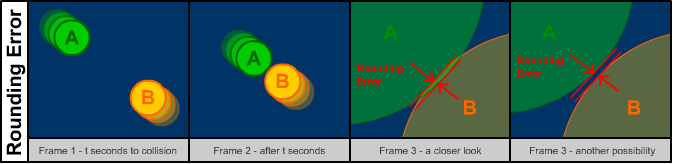Figure 3 - Rounding Error

Since the second problem is easy to fix, lets address it first. In order to catch all the collisions, we simply
look for all balls that are 'close enough' together. We define that two balls are 'close enough' if they are at
least epsilon apart where epsilon is some small constant. Since the rounding error is very small (around 10^-14),
our epsilon can also be quite small. A value like 10^-9 will work just fine. So now, rather than testing if the
distance between the centers of the two circles is the sum of their radii, we check if it is at most the sum of
their radii plus the value of epsilon. If the distance is less than this value, then we assume a collision has
occured.

The first problem is slightly more difficult to fix. A knowledge of vectors and the dot product simplify the
problem a great deal. Before checking for a collision between two balls, we simply check if the two balls are
moving towards each other. If they aren't then we know no collision will occur. Not only will this fix the
sticking problem, but it will also speed up the program because checking if two balls are moving towards each
other is a lot easier than checking if they are colliding. Heck, we can also add this check before finding the
time to collision between two balls.

To determine if two balls are moving towards each other, we first need to find the velocity of one ball relative
to the velocity of the other ball. Lets say we have two balls called A and B. To find the velocity of A relative
to B, we subtract B's velocity from A (keeping in mind that the velocity is a vector quantity). In component form,
the relative velocity would be described like so: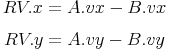Now we need to calculate the vector from the position of A to the position of B: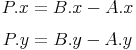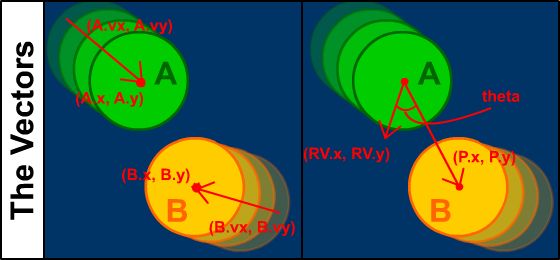Figure 4 - The Vectors

The dot product states that: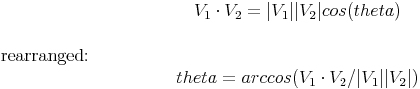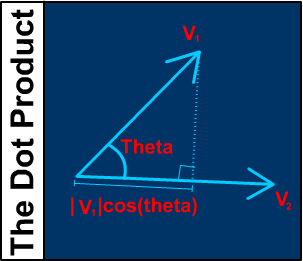Figure 5 - The Dot Product

And what are the vectors V_1 and V_2? In our case, they are the vectors we defined earlier, namely the vector that
represents the relative velocity of A with respect to B (RV) and the vector between the positions of A and B (P).
If theta is an acute angle, then the balls are moving towards each other. Theta is acute only when the expression
in the arccos function is positive. Since the denominator in that expression is always positive, all we need to do
is check if the value of A dot B is positive. If A and B are two vectors (in two dimensions) then: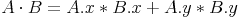With all this knowledge, we can now write a function that returns a boolean to tell us whether two balls are
moving towards each other:

 Turing: /* Tells us if two balls are moving towards each other */ fcn movingToBall (b1, b2 : BallData) : boolean     /* Position Vector dotted with the Relative Velocity Vector */     result (b2.x - b1.x) * (b1.vx - b2.vx) + (b2.y - b1.y) * (b1.vy - b2.vy) > 0 end movingToBall

Listing 3 - A Function Which Tells Us If Two Balls Are Moving Towards Each Other

Putting everything together and adding many random balls with some collision reaction we get the following code:

 Turing: View.Set ("offscreenonly,graphics:300;300") type BallData :     record         x, y, vx, vy, vxp, vyp, r, m : real     end record /* The amount of delay between frames */ const DELAY := 50 /* The maximum distance two balls can be apart and still be considered colliding */ const EPSILON := 1e-9 /* The number of balls */ const NUMBALLS := 5 /* We anticipate many balls so we create an array */ var balls : array 1 .. NUMBALLS of BallData /* Stores the amount of time untill a collision occurs */ var t : real /* Initialize the balls */ for i : 1 .. NUMBALLS     balls (i).x := Rand.Int (1, maxx)     balls (i).y := Rand.Int (1, maxy)     balls (i).vx := Rand.Int (-5, 5)     balls (i).vy := Rand.Int (-5, 5)     balls (i).vxp := balls (i).vx     balls (i).vyp := balls (i).vy     balls (i).r := Rand.Int (15, 30)     balls (i).m := Math.PI * balls (i).r ** 2 end for /* Tells us if two balls are moving towards each other */ fcn movingToBall (b1, b2 : BallData) : boolean     /* Position Vector dotted with the Relative Velocity Vector */     result (b2.x - b1.x) * (b1.vx - b2.vx) + (b2.y - b1.y) * (b1.vy - b2.vy) > 0 end movingToBall /* Returns the amount of frames untill a collision will occur */ fcn timeToCollision : real     var t : real := maxint     var A, B, C, D, DISC : real     /* Loop through every pair of balls and calculate when they will collide */     for i : 1 .. upper (balls)         for j : i + 1 .. upper (balls)             if movingToBall (balls (i), balls (j)) then                 /* Breaking down the formula for t */                 A := balls (i).vx ** 2 + balls (i).vy ** 2 - 2 * balls (i).vx * balls (j).vx + balls (j).vx ** 2 - 2 * balls (i).vy * balls (j).vy + balls (j).vy ** 2                 B := -balls (i).x * balls (i).vx - balls (i).y * balls (i).vy + balls (i).vx * balls (j).x + balls (i).vy * balls (j).y + balls (i).x * balls (j).vx -                     balls (j).x * balls (j).vx + balls (i).y * balls (j).vy - balls (j).y * balls (j).vy                 C := balls (i).vx ** 2 + balls (i).vy ** 2 - 2 * balls (i).vx * balls (j).vx + balls (j).vx ** 2 - 2 * balls (i).vy * balls (j).vy + balls (j).vy ** 2                 D := balls (i).x ** 2 + balls (i).y ** 2 - balls (i).r ** 2 - 2 * balls (i).x * balls (j).x + balls (j).x ** 2 - 2 * balls (i).y * balls (j).y +                     balls (j).y ** 2 - 2 * balls (i).r * balls (j).r - balls (j).r ** 2                 DISC := (-2 * B) ** 2 - 4 * C * D                 /* If the discriminent if non negative, a collision will occur and *                  * we must compare the time to our current time of collision. We   *                  * udate the time if we find a collision that has occurd earlier   *                  * than the previous one.                                          */                 if DISC >= 0 then                     /* We want the smallest time */                     t := min (min (t, 0.5 * (2 * B - sqrt (DISC)) / A), 0.5 * (2 * B + sqrt (DISC)) / A)                 end if             end if         end for     end for     result t end timeToCollision /* Draws all the balls to the screen */ proc drawBalls     for i : 1 .. upper (balls)         Draw.Oval (round (balls (i).x), round (balls (i).y), round (balls (i).r), round (balls (i).r), black)     end for end drawBalls /* Updates all the balls attributes. If a collision Occures in between frames, *  * the balls will be updated to the point of the collision. We return when the *  * collision occurs so that we can adjust the delay in the main loop.          */ fcn updateBalls : real     /* We want to increment by at most one frame */     var t := min (1, timeToCollision)     for i : 1 .. upper (balls)         balls (i).x += balls (i).vx * t         balls (i).y += balls (i).vy * t     end for     result t end updateBalls /* Collision reaction function */ proc collide (var b1, b2 : BallData)     var nx := (b1.x - b2.x) / (b1.r + b2.r)     var ny := (b1.y - b2.y) / (b1.r + b2.r)     var a1 := b1.vx * nx + b1.vy * ny     var a2 := b2.vx * nx + b2.vy * ny     var p := 2 * (a1 - a2) / (b1.m + b2.m)     b1.vxp := b1.vx - p * nx * b2.m     b1.vyp := b1.vy - p * ny * b2.m     b2.vxp := b2.vx + p * nx * b1.m     b2.vyp := b2.vy + p * ny * b1.m end collide /* Checks if a collision has occured between any of the balls */ proc checkForCollision     for i : 1 .. upper (balls)         for j : i + 1 .. upper (balls)             if movingToBall (balls (i), balls (j)) and (balls (j).x - balls (i).x) ** 2 + (balls (j).y - balls (i).y) ** 2 <= (balls (i).r + balls (j).r + EPSILON) ** 2 then                 collide (balls (i), balls (j))             end if         end for         if balls (i).x < 1 or balls (i).x > maxx then             balls (i).vxp *= -1         end if         if balls (i).y < 1 or balls (i).y > maxy then             balls (i).vyp *= -1         end if     end for     for i : 1 .. upper (balls)         balls (i).vx := balls (i).vxp         balls (i).vy := balls (i).vyp     end for end checkForCollision /* Main animation loop */ loop     drawBalls     checkForCollision     t := updateBalls     View.Update     cls     /* If a collision occurs in between frames, we have to use a shorter delay *      * to keep the animation speed consistent                                  */     Time.DelaySinceLast (round (DELAY * t)) end loop

Listing 4 - Putting Everything Together

Note that the sticking phenominon may occur with the balls against the walls. To fix this, use the same principals
we used with the balls sticking to each other problem. That is, find the time at which the first collision will
occur between a ball and a wall and update the animation to that time. Also, make sure that the ball is moving
towards the wall like we did before. This is much easier than checking if two balls are moving towards each other
because we just need to see what direction the ball is moving in (ie if the balls x velocity is negative, it will
is moving towards the right wall etc...)

I hope that you have enjoyed this tutorial. If there is a large demand, I may follow up this tutorial with a
collision response tutorial. Thanks for reading!Posted: Wed Jan 17, 2007 11:44 pm   Post subject: RE:Perfect Circle - Circle Collision Detection 3

wow.. amazing tutorial. very well done, even though you lost me at the dot products.. my math isn't advanced enough.

i would really like to see your take

also, i've tried to get into part one of your circle collision detection tutorial but the link doesn't seem to work.. it's weird cuz i tried it many times now, and it just freezes after loading a few of the images at the top.
screenshot:
http://img251.imageshack.us/img251/8771/01172007214425gs2.jpg

EDIT: part 2 does the same thing.

screenshot:
http://img265.imageshack.us/img265/2823/01172007214602ni4.jpgClaytonPosted: Thu Jan 18, 2007 12:04 am   Post subject: Re: Perfect Circle - Circle Collision Detection 3

Those aren't seperate parts, but actually the same tutorial. zylum had issues posting it as it was too long for one post (that's why it freezes up), so he posted another with seperate posts so as to let it be viewed evenrollerdudePosted: Mon Apr 30, 2007 12:30 pm   Post subject: Re: Perfect Circle - Circle Collision Detection

WOOT!!

awsome... for me to think about how to do that would take a great deal of time and end up not working anyway

+10 bits
+1 karma

from me!rollerdudePosted: Mon Apr 30, 2007 12:35 pm   Post subject: Re: Perfect Circle - Circle Collision Detection

since the space is frictionless (and im sure it doesn't really relate to this post) does that mean that the sums of the speeds of the balls remain constant?rollerdudePosted: Mon Apr 30, 2007 12:44 pm   Post subject: Re: Perfect Circle - Circle Collision Detection

and i dont mean to be a nag... but how come the balls halfway leave the screen?Posted: Tue May 01, 2007 9:09 am   Post subject: Re: Perfect Circle - Circle Collision Detection

Thanks Zylum, this is exactly what i needed (although the math seems a litle advanced for me, ill figure it out though).

+ Karma!

Oh, and rollerdude, the reason the balls tend to go halfway out of the screen because Zylum used the origin of the circle (the centre point) for the wall collision detection. Don't know why he/she did it that way, but that can be easily fixed just by checking to see if the distance between the radius of the circle and the wall is smaller than the radius + epsilon (a new thing for me, thans Zylum)

As for the balls remaining at a constant speed when they collide, it depends on how directly they hit each other, as well as the direciton and velocity. But you are right, since the space is frictionless, the sum of the velocities of the balls will remain the same, since their energy is only being passed around with each other. If friction were to be added (in a pool game), eventually the energy of the balls would eventually be spent due to friction.zylumPosted: Tue May 01, 2007 12:46 pm   Post subject: RE:Perfect Circle - Circle Collision Detection

im gald you guys enjoyed it and im also glad to see some feed backbelieve it or not it takes a while to come up with a tutorial like that and not getting too many comments is sort of discouraging and i dont have as much motivation to write up new tutorials. so if you have read any tutorials and enjoyed them make sure you leave a comment! im sure other tutorial writers feel the same.DaetyrnisPosted: Fri May 04, 2007 6:24 pm   Post subject: RE:Perfect Circle - Circle Collision Detection

That was a really good tutorial. ^^

*thumbs way up*Warchamp7Posted: Fri May 11, 2007 11:09 am   Post subject: RE:Perfect Circle - Circle Collision Detection

Very nice job, pretty cool and easy to tinker with+KarmaReality CheckPosted: Wed May 30, 2007 7:57 am   Post subject: Re: Perfect Circle - Circle Collision Detection

Hey. Great tutorial. Its helped me A LOT. I have just one question though. In your "movingToBall" function, after doing the calculation and everything why do you put "> 0"? Does it return a false if its less than 0?richcashPosted: Wed May 30, 2007 12:20 pm   Post subject: Re: Perfect Circle - Circle Collision Detection

Reality Check wrote:

Hey. Great tutorial. Its helped me A LOT. I have just one question though. In your "movingToBall" function, after doing the calculation and everything why do you put "> 0"? Does it return a false if its less than 0?

Yes, it returns a boolean. The function returns false if the dot product of the position vector and the relative velocity vector is less than or equal to 0. It returns true otherwise, which means the balls are moving toward each other (which is a method I've never heard of before but is VERY cool! Thanks for that zylum.)

@Reality Check : Maybe you'll get this format bettter :
 Turing: fcn movingToBall (b1, b2 : BallData) : boolean     var dotProd := (b2.x - b1.x) * (b1.vx - b2.vx) + (b2.y - b1.y) * (b1.vy - b2.vy)     if dotProd > 0 then         result true %balls moving toward each other     else         result false end movingToBallPosted: Sun Jun 17, 2007 7:18 pm   Post subject: RE:Perfect Circle - Circle Collision Detection

God damn thats complicated.

A guy a know just finds the equation of the line between the position of two objects from the current frame, and the next frame, and finds the point of intersection between those two lines...

sounds easyer, would it be less accurate?A.JPosted: Sun Mar 02, 2008 12:07 pm   Post subject: Re: Perfect Circle - Circle Collision Detection

 code: if balls (i).x < 1 or balls (i).x > maxx then             balls (i).vxp *= -1         end if         if balls (i).y < 1 or balls (i).y > maxy then             balls (i).vyp *= -1         end if

to check for collision with the wall, we can do this :
 code: if balls (i).x < balls (i).r or balls (i).x > maxx - balls (i).r then             balls (i).vxp *= -1         end if         if balls (i).y < balls (i).r or balls (i).y > maxy - balls (i).r then             balls (i).vyp *= -1         end if

to make it more exactDisplay posts from previous: All Posts1 Day7 Days2 Weeks1 Month3 Months6 Months1 Year Oldest FirstNewest FirstPage 1 of 3  [ 34 Posts ]
Goto page 1, 2, 3  Next
 Jump to:  Select a forum  CompSci.ca ------------ - Network News - General Discussion     General Forums   -----------------   - Hello World   - Featured Poll   - Contests     Contest Forums   -----------------   - DWITE   - [FP] Contest 2006/2008   - [FP] 2005/2006 Archive   - [FP] 2004/2005 Archive   - Off Topic     Lounges   ---------   - User Lounge   - VIP Lounge     Programming -------------- - General Programming     General Programming Forums   --------------------------------   - Functional Programming   - Logical Programming   - C     C   --   - C Help   - C Tutorials   - C Submissions   - C++     C++   ----   - C++ Help   - C++ Tutorials   - C++ Submissions   - Java     Java   -----   - Java Help   - Java Tutorials   - Java Submissions   - Ruby     Ruby   -----   - Ruby Help   - Ruby Tutorials   - Ruby Submissions   - Turing     Turing   --------   - Turing Help   - Turing Tutorials   - Turing Submissions   - PHP     PHP   ----   - PHP Help   - PHP Tutorials   - PHP Submissions   - Python     Python   --------   - Python Help   - Python Tutorials   - Python Submissions   - Visual Basic and Other Basics     VB   ---   - Visual Basic Help   - Visual Basic Tutorials   - Visual Basic Submissions     Education ----------- - Student Life   Graphics and Design ----------------------- - Web Design     Web Design Forums   ---------------------   - (X)HTML Help   - (X)HTML Tutorials   - Flash MX Help   - Flash MX Tutorials   - Graphics     Graphics Forums   ------------------   - Photoshop Tutorials   - The Showroom   - 2D Graphics   - 3D Graphics     Teams ------ - dTeam Public

 Style: Appalachia blueSilver eMJay subAppalachia subBlue subCanvas subEmjay subGrey subSilver subVereor Search: